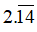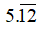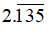# SSC Worksheet for Chapter - 1 Numbers Class 8

## Find SSC Worksheet for chapter-1 Numbers class 8

CLASS-8

BOARD: SSC

Mathematic Worksheet - 1

TOPIC: Numbers

For otherSSC Worksheet for class 8 Mathematiccheck out main page of Physics Wallah.

1. Which of the following is a terminating recurring decimal ?
1. 3.125
2.3. 7.88…
4. 4.818...
2. Which of the following is a terminating recurring decimal ?
1.2. 23.18245
3. 23.18245
4. 7.33...
3. Which of the following is a non-terminating recurring decimal ?
1. 5.666…
2. 8.676
3. 8.555
4. 8.8924
4. Which of the following a non-terminating recurring decimal?
1.2. 5.12
3. 5.1212
4. 5.1021
5. Which of the following is a rational number ?
1. 2.135135...
2. 2.1313...
3. 2.11...
4.6. Which of the following is rational number ?
1. √5
2. √4
3. √2
4. √3
7. Which of the following is an irrational number ?
1. √2
2. √25
3. √64
4. √3
8. Which of the following is an irrational number ?
1. 8.92
2. √17
3. 3.1414
4. √121
9. -28/5 is a
1. Natural number
2. Rational number
3. Integer number
4. Irrational number
10. √ 8is a
1. Natural number
2. Whole number
3. Rational number
4. Irrational number
11. 7/2is a
1. Natural number
2. Irrational number
3. Rational number
4. Real number
12. Find the square toot of 252.3419 by the division method.
13. Find the square root of 25.25 by the division method.

### Solutions: to worksheet-1 Topic-Numbers

 1. (a) 2. (c) 3. (a) 4. (a) 5. (d) 6. (b) 7. (a) 8. () 9. (b) 10. (d) 11. (b, d